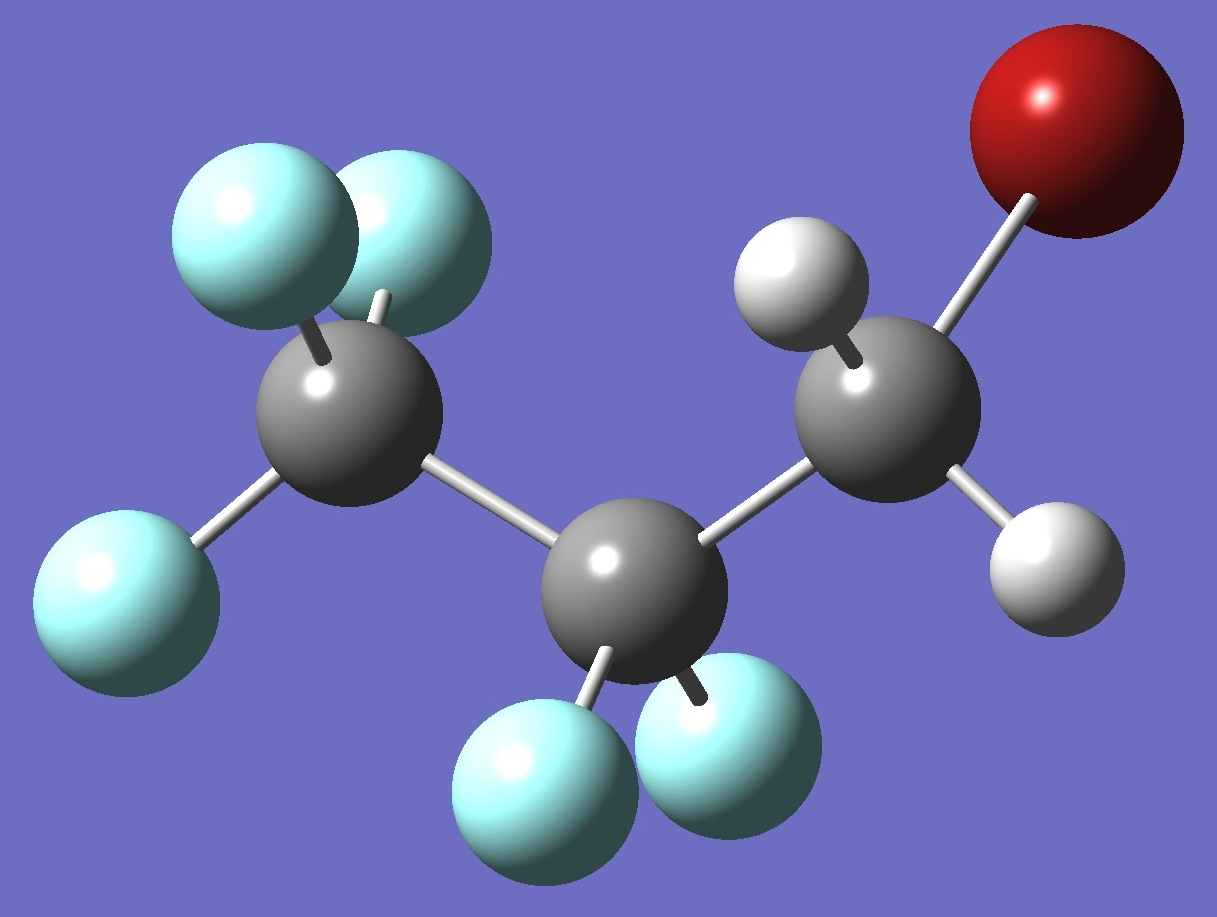CF3CF2CH2BrBromine

Nuclear Quadrupole Coupling Constants

in gauche-3-Bromo-1,1,1,2,2-Pentafluoropropane

Calculation of the Br nqcc's in gauche-3-bromo-1,1,1,2,2-pentafluoropropane was made here on a molecular structure (ropt) derived by MP2/6-311+G(3df,3pd) optimization, and on this same structure but with empirically corrected bond lengths (~ re) as described here.  Calculated nqcc's are given in Tables 1 and 2.  Structure parameters are given in Z-matrix format in Table 3, rotational constants in Table 4.

In Tables 1 and 2, subscripts a,b,c refer to the principal axes of the inertia tensor; x,y,z to the principal axes of the nqcc tensor.  ETA = (Xxx - Xyy)/Xzz.  Ø (degrees) is the angle between its subscripted parameters.

RMS is the root mean square difference between calculated and experimental diagonal nqcc's (percentage of the average of the magnitudes of the experimental nqcc's).  RSD is the calibration residual standard deviation of the B1LYP/TZV(3df,3p) model for calculation of the bromine efg's/nqcc's.

 Table 1.  79Br nqcc's in g-CF3CF2CH2Br (MHz).  Calculation was made on the (1) ropt and (2) ~ re structures. Calc. (1) Calc. (2) Expt. Xaa 230.65 232.43 Xbb - 58.13 - 59.20 Xcc - 172.52 - 173.22 Xab 371.99 372.48 Xac 268.63 269.16 Xbc 189.96 189.56 RMS RSD 1.58 (0.39 %) 1.58 (0.39 %) Xxx - 305.75 - 306.46 Xyy - 315.83 - 316.30 Xzz 621.58 622.76 ETA 0.0162 0.0158 Øz,CBr 1.41 1.40

 Table 2.   81Br nqcc's in g-CF3CF2CH2Br (MHz).  Calculation was made on the (1) ropt and (2) ~ re structures. Calc. (1) Calc. (2) Expt. Xaa 193.56 195.04 Xbb - 49.41 - 50.30 Xcc - 144.15 - 144.74 Xab 310.47 310.88 Xac 224.63 225.08 Xbc 158.39 158.06 RMS RSD 1.38 (0.40 %) 1.38 (0.40 %)

 Table 3.  g-CF3CF2CH2Br   MP2/6-311+G(3df,3pd) Structure Parameters (Å and degrees).  Approximate equilibrium (~ re) bond lengths are given in parentheses.C               C,1,B1  C,2,B2,1,A1  H,3,B3,2,A2,1,D1,0  H,3,B4,2,A3,1,D2,0  Br,3,B5,2,A4,1,D3,0  F,2,B6,2,A5,3,D4,0  F,2,B7,1,A6,3,D5,0  F,1,B8,2,A7,3,D6,0  F,1,B9,2,A8,3,D7,0  F,1,B10,2,A9,3,D8,0 B1=1.54331146       (1.5401)    B2=1.51243048    B3=1.08577619    B4=1.08446299    B5=1.92066844        (1.9242)    B6=1.34425986        (1.3428)    B7=1.35703865        (1.3554)    B8=1.32678675        (1.3257)    B9=1.32496207        (1.3240)    B10=1.33373370      (1.3325)    A1=116.27063822    A2=106.77264044    A3=109.33122259    A4=114.16512766    A5=107.83249381    A6=106.12278811    A7=109.79170759    A8=111.42101947    A9=109.37219184    D1=173.49511920    D2=54.47082858    D3=-67.36416342    D4=-126.24363919    D5=118.22303390    D6=-167.61028693    D7=72.00250004    D8=-48.38558339

 Table 4.  g-CF3CF2CH2Br   Rotational Constants (MHz).  79Br Species. ropt ~ re Expt. A 1919.07 1924.4 B 732.6 732.9 C 659.3 659.8

F.E.Marshall, N.Moon, T.D.Persinger, D.J.Gillcrist, N.E.Shreve, W.C.Bailey, and G.S.Grubbs II, Abstract FB05, 72th International Symposium on Molecular Spectroscopy, Champaign-Urbana, 2017.

t-CF3CF2CH2Br CF3CF2Br CH3CH2Br

Molecules/Bromine

gCF3CF2CH2Br.html